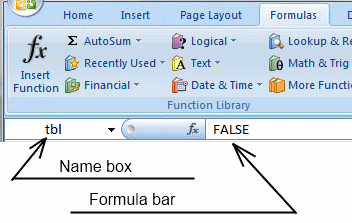Author: Oscar Cronquist Article last updated on November 27, 2018

### Question:

How do I create a new unique distinct list from a column. I also want the list sorted from large to small by the number of occurrances?

=INDEX(\$B\$3:\$B\$14, MATCH(LARGE(IF(COUNTIF(\$D\$2:D2, \$B\$3:\$B\$14)=0, COUNTIF(\$B\$3:\$B\$14, \$B\$3:\$B\$14), ""), 1), COUNTIF(\$B\$3:\$B\$14, \$B\$3:\$B\$14)*(COUNTIF(\$D\$2:D2, \$B\$3:\$B\$14)=0), 0))

How to create an array formula

1. Copy (Ctrl + c) and paste (Ctrl + v) array formula into formula bar. See picture below.
2. Press and hold Ctrl + Shift.
3. Press Enter once.
4. Release all keys.Recommended article

A beginners guide to Excel array formulas

Array formulas allows you to do advanced calculations not possible with regular formulas.

How to copy array formula in cell D3

1. Select cell D3
2. Copy cell (Ctrl + c)
3. Select cell range D4:D8
4. Paste (Ctrl + v)

Formula in E3:

=COUNTIF(\$B\$3:\$B\$14, D3)

How to use the COUNTIF function

Counts the number of cells that meet a specific condition.

Explaining array formula in cell D3

Step 1 - Remove duplicates

=INDEX(List, MATCH(LARGE(IF(COUNTIF(\$D\$2:D2, List)=0, COUNTIF(List, List), ""), 1), COUNTIF(List, List)*(COUNTIF(\$D\$2:D2, List)=0), 0))

IF(COUNTIF(\$D\$2:D2, List)=0, COUNTIF(List, List), "")

becomes

IF(COUNTIF("List sorted by occurrances", {"DD";"AA";"AA";"BB";"AA"; "DD";"EE";"AA";"BB";"EE";"CC";"EE"})=0, COUNTIF({"DD";"AA";"AA";"BB";"AA"; "DD";"EE";"AA";"BB";"EE";"CC";"EE"}, {"DD";"AA";"AA";"BB";"AA"; "DD";"EE";"AA";"BB";"EE";"CC";"EE"}), "")

becomes

IF({0;0;0;0;0;0;0;0;0;0;0;0}=0, {2;4;4;2;4;2;3;4;2;3;1;3}, "")

becomes

IF({TRUE;TRUE;TRUE;TRUE;TRUE; TRUE;TRUE;TRUE;TRUE;TRUE;TRUE;TRUE}, {2;4;4;2;4;2; 3;4;2;3;1;3}, "")

and returns

{2;4;4;2;4;2;3;4;2;3;1;3}

How to use the IF function

Checks if a logical expression is met. Returns a specific value if TRUE and another specific value if FALSE.

How to use the COUNTIF function

Counts the number of cells that meet a specific condition.

Step 2 - Find maximum value

=INDEX(List, MATCH(LARGE(IF(COUNTIF(\$D\$2:D2, List)=0, COUNTIF(List, List), ""), 1), COUNTIF(List, List)*(COUNTIF(\$D\$2:D2, List)=0), 0))

LARGE(IF(COUNTIF(\$D\$2:D2, List)=0, COUNTIF(List, List), ""), 1)

becomes

LARGE({2;4;4;2;4;2;3;4;2;3;1;3}, 1)

and returns 4.

How to use the SMALL function

The SMALL function lets you extract a number in a cell range based on how small it is compared to the other numbers in the group.

Step 3 - Return the relative position of an item in an array that matches a specified value

=INDEX(List, MATCH(LARGE(IF(COUNTIF(\$D\$2:D2, List)=0, COUNTIF(List, List), ""), 1), COUNTIF(List, List)*(COUNTIF(\$D\$2:D2, List)=0), 0))

MATCH(LARGE(IF(COUNTIF(\$D\$2:D2, List)=0, COUNTIF(List, List), ""), 1), COUNTIF(List, List)*(COUNTIF(\$D\$2:D2, List)=0), 0)

becomes

MATCH(4, COUNTIF(List, List)*(COUNTIF(\$D\$2:D2, List)=0), 0)

becomes

MATCH(4, {2;4;4;2;4;2;3;4;2;3;1;3}), 0)

and returns 2.

How to use the MATCH function

Identify the position of a value in an array.

Step 4 - Return a value in a specific position in a given range

=INDEX(List, MATCH(LARGE(IF(COUNTIF(\$D\$2:D2, List)=0, COUNTIF(List, List), ""), 1), COUNTIF(List, List)*(COUNTIF(\$D\$2:D2, List)=0), 0))

becomes

=INDEX(List, 2)

becomes

=INDEX({"DD";"AA";"AA";"BB";"AA";"DD"; "EE";"VV";"BB";"EE";"VV";"EE"}, 2)

and returns "AA".

How to use the INDEX function

Gets a value in a specific cell range based on a row and column number.

### Get excel example file

unique-sorted-by-occurances.xls
(Excel 97-2003 Workbook *.xls)